### Home > MC2 > Chapter 5 > Lesson 5.3.1 > Problem5-90

5-90.

Decide if these angles are acute, obtuse, or right angles. All diagrams are drawn to scale. Homework Help ✎

 a.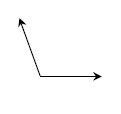b.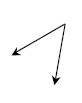c.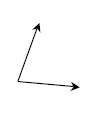d.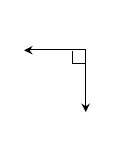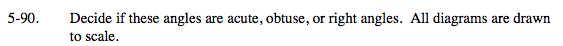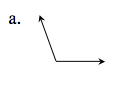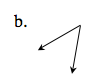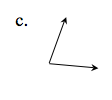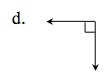Acute angle: An angle with a measure between (but not including) 0° and 90°.
Right angle: An angle measuring 90°.
Obtuse angle: An angle with a measure between (but not including) 90° an 180°.

To determine the type of angle, use a 90° angle as a reference point.

Since the angle is greater than 90°, it is obtuse.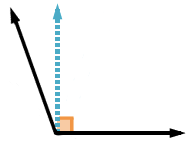Acute angle